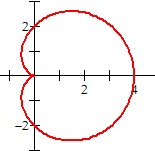index: click on a letter A B C D E F G H I J K L M N O P Q R S T U V W X Y Z A to Z index index: subject areas numbers & symbols sets, logic, proofs geometry algebra trigonometry advanced algebra & pre-calculus calculus advanced topics probability & statistics real world applications multimedia entrieswww.mathwords.com about mathwords website feedback

Cardioid

A curve that is somewhat heart shaped. A cardioid can be drawn by tracing the path of a point on a circle as the circle rolls around a fixed circle of the same radius. The equation is usually written in polar coordinates.

Note: A cardioid is a special case of the limaçon family of curves.

Cardioid:   r = a ± a cos θ (horizontal) or  r = a ± a sin θ (vertical)r = 2 + 2 cos θr = 2 + 2 sin θ